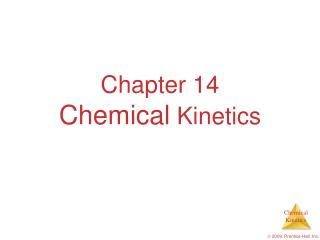DownloadDownload PresentationChapter 14 Chemical Kinetics

# Chapter 14 Chemical Kinetics

Download Presentation## Chapter 14 Chemical Kinetics

- - - - - - - - - - - - - - - - - - - - - - - - - - - E N D - - - - - - - - - - - - - - - - - - - - - - - - - - -
##### Presentation Transcript

1. Chapter 14Chemical Kinetics  2009, Prentice-Hall, Inc.

2. Rate Laws • A rate law shows the relationship between the reaction rate and the concentrations of reactants. • The exponents tell the order of the reaction with respect to each reactant. • Since the rate law is Rate = k [NH4+] [NO2−] the reaction is First-order in [NH4+] and First-order in [NO2−].  2009, Prentice-Hall, Inc.

3. Rate Laws Rate = k [NH4+] [NO2−] • The overall reaction order can be found by adding the exponents on the reactants in the rate law. • This reaction is second-order overall.  2009, Prentice-Hall, Inc.

4. = −kt ln [A]t [A]0 Integrated Rate Laws Using calculus to integrate the rate law for a first-order process gives us Where [A]0 is the initial concentration of A, and [A]t is the concentration of A at some time, t, during the course of the reaction.  2009, Prentice-Hall, Inc.

5. = −kt ln [A]t [A]0 Integrated Rate Laws Manipulating this equation produces… ln [A]t− ln [A]0 = −kt ln [A]t = −kt + ln [A]0 …which is in the form y = mx + b  2009, Prentice-Hall, Inc.

6. First-Order Processes ln [A]t = -kt + ln [A]0 Therefore, if a reaction is first-order, a plot of ln [A] vs. t will yield a straight line, and the slope of the line will be -k.  2009, Prentice-Hall, Inc.

7. CH3NC CH3CN First-Order Processes Consider the process in which methyl isonitrile is converted to acetonitrile.  2009, Prentice-Hall, Inc.

8. CH3NC CH3CN First-Order Processes This data was collected for this reaction at 198.9 °C.  2009, Prentice-Hall, Inc.

9. First-Order Processes • When ln P is plotted as a function of time, a straight line results. • Therefore, • The process is first-order. • k is the negative of the slope: 5.1  10-5 s−1.  2009, Prentice-Hall, Inc.

10. 1 [A]0 1 [A]t = kt + Second-Order Processes Similarly, integrating the rate law for a process that is second-order in reactant A, we get also in the form y = mx + b  2009, Prentice-Hall, Inc.

11. 1 [A]0 1 [A]t = kt + 1 [A] Second-Order Processes So if a process is second-order in A, a plot of vs. t will yield a straight line, and the slope of that line is k.  2009, Prentice-Hall, Inc.

12. NO2(g) NO (g) + O2(g) 1 2 Second-Order Processes The decomposition of NO2 at 300°C is described by the equation and yields data comparable to this:  2009, Prentice-Hall, Inc.

13. Second-Order Processes • Plotting ln [NO2] vs.t yields the graph at the right. • The plot is not a straight line, so the process is not first-order in [A].  2009, Prentice-Hall, Inc.

14. 1 [NO2] Second-Order Processes • Graphing ln vs. t, however, gives this plot. • Because this is a straight line, the process is second-order in [A].  2009, Prentice-Hall, Inc.

15. Half-Life • Half-life is defined as the time required for one-half of a reactant to react. • Because [A] at t1/2 is one-half of the original [A], [A]t = 0.5 [A]0.  2009, Prentice-Hall, Inc.

16. 0.5 [A]0 [A]0 ln = −kt1/2 0.693 k = t1/2 Half-Life For a first-order process, this becomes ln 0.5 = −kt1/2 −0.693 = −kt1/2 NOTE: For a first-order process, then, the half-life does not depend on [A]0.  2009, Prentice-Hall, Inc.

17. 1 k[A]0 1 [A]0 1 [A]0 1 [A]0 2 [A]0 1 0.5 [A]0 2 − 1 [A]0 = kt1/2 + = kt1/2 + = = kt1/2 = t1/2 Half-Life For a second-order process,  2009, Prentice-Hall, Inc.

18. Temperature and Rate • Generally, as temperature increases, so does the reaction rate. • This is because k is temperature dependent.  2009, Prentice-Hall, Inc.

19. The Collision Model • In a chemical reaction, bonds are broken and new bonds are formed. • Molecules can only react if they collide with each other.  2009, Prentice-Hall, Inc.

20. The Collision Model Furthermore, molecules must collide with the correct orientation and with enough energy to cause bond breakage and formation.  2009, Prentice-Hall, Inc.

21. Activation Energy • In other words, there is a minimum amount of energy required for reaction: the activation energy, Ea. • Just as a ball cannot get over a hill if it does not roll up the hill with enough energy, a reaction cannot occur unless the molecules possess sufficient energy to get over the activation energy barrier.  2009, Prentice-Hall, Inc.